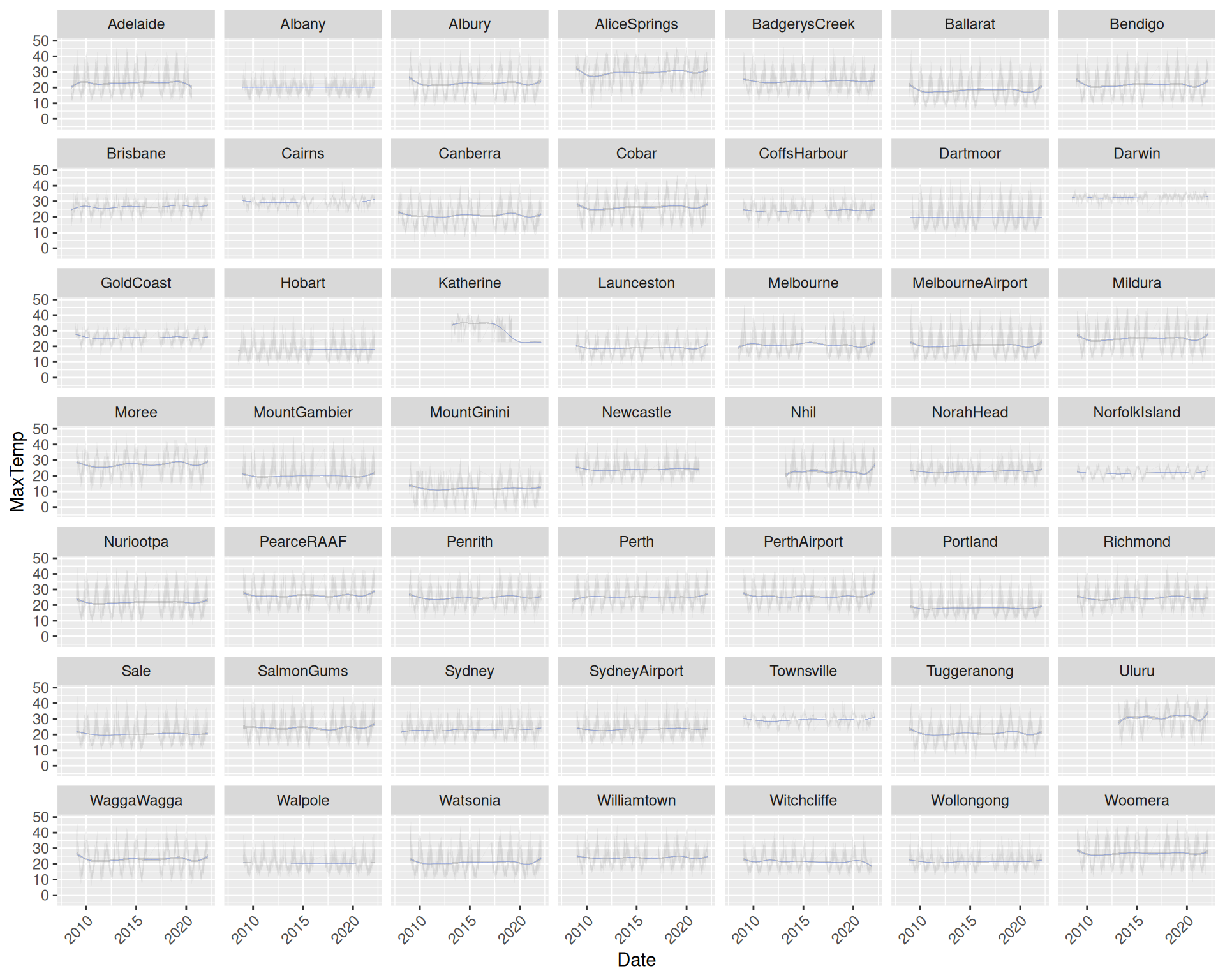## 11.27 Faceted Location Thin Lines

20200608ds %>%
ggplot(aes(x=date, y=max_temp)) +
geom_line(alpha=0.1, size=0.05) +
geom_smooth(method="gam", formula=y~s(x, bs="cs"), size=0.05) +
facet_wrap(~location) +
theme(axis.text.x=element_text(angle=45, hjust=1)) +
labs(x=vnames["date"], y=vnames["max_temp"])

An alternative is to present the plot as a line chart rather than a scatter plot. It does make more sense for a time series plot such as this, though the effect is little changed due to the amount of data being displayed.

Changing to lines simply uses ggplot2::geom_line() instead of ggplot2::geom_point(). Very thin lines are used as specified through the size= option. Nonetheless, the data remains quite dense.

Your donation will support ongoing development and give you access to the PDF version of this book. Desktop Survival Guides include Data Science, GNU/Linux, and MLHub. Books available on Amazon include Data Mining with Rattle and Essentials of Data Science. Popular open source software includes rattle, wajig, and mlhub. Hosted by Togaware, a pioneer of free and open source software since 1984.